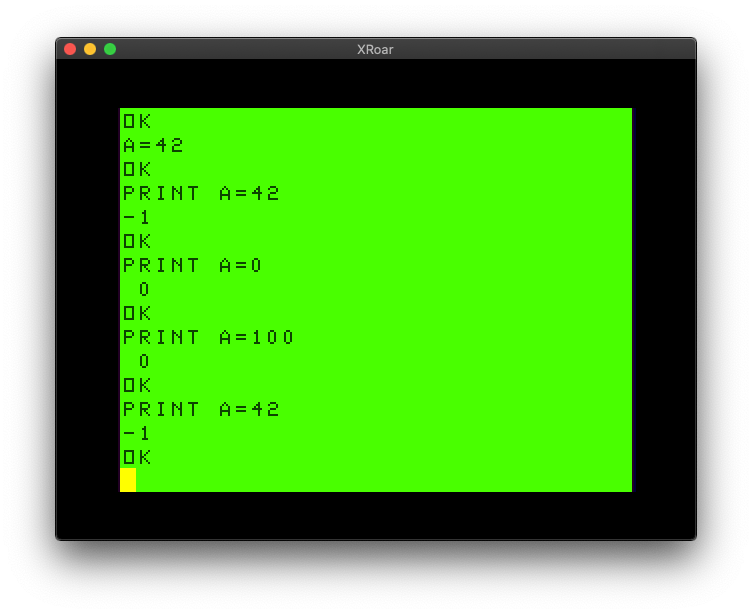# IF AND/OR THEN versus IF THEN IF

## Or, “I’ll NEVER compare multiple values the same way again”

Here is a quick Optimizing Color BASIC quickie.

Tonight, I saw this YouTube video from Robin at 8-Bit Show and Tell. He was responding to someone who noticed a bit of code in an old Commodore magazine that did:

`IF Z>192 THEN IF Z<219 THEN Z=Z-128`

`IF Z>192 AND Z<219 THEN Z=Z-128`

He performed a benchmark very much like the ones I have been doing on the CoCo (I’m glad I’m not the only one doing this). I decided to try it on the CoCo and see what happened here.

I first tried the normal “IF AND THEN” way:

```0 REM IFAND.BAS
5 DIM TE,TM,B,A,TT
10 FORA=0TO3:TIMER=0:TM=TIMER
20 FORB=0TO1000
30 IF Z>192 AND Z<219 THEN Z=Z-128
70 NEXT
80 TE=TIMER-TM:PRINT,TE
90 TT=TT+TE:NEXT:PRINTTT/A:END
```

This produced 875 on my Xroar emulator.

Then I tried the “odd” IF AND THEN way…

```0 REM THENIF.BAS
5 DIM TE,TM,B,A,TT
10 FORA=0TO3:TIMER=0:TM=TIMER
20 FORB=0TO1000
30 IF Z>192 THEN IF Z<219 THEN Z=Z-128
70 NEXT
80 TE=TIMER-TM:PRINT,TE
90 TT=TT+TE:NEXT:PRINTTT/A:END```

This produced 472! A significant time savings just by changing the way you compare multiple things.

Thinking this through, I have some theories, based on some very inefficient C code I found in a work project once.

See A C tale of two ampersands for that story…

Could BASIC be doing the same thing?

## IF THEN BASIC

“IF this AND that THEN” surely isn’t doing a logical AND for values, is it? It might be, since you can print a comparison like this:

In Color BASIC, true is -1 and false is 0. So perhaps doing this:

`IF A=1 AND B=2 THEN PRINT "BOTH ARE TRUE"`

…is actually turning into…

`IF -1 AND 0 THEN PRINT "BOTH ARE TRUE"`

…with -1 or 0 being used based on the conditions. Let’s try:

Oh my. Maybe it IS doing this, thus requiring the values to be saved and compared, making it slower just like C generating more code to do the same thing.

I know I could just look at the disassembly in Color BASIC Unravelled and know exactly what is going on, but it’s more fun to experiment. How can we test this?

Well, things that return true/false return -1 or 0. You can AND two numbers together and get mathematical results. Even a compare like:

`IF A\$="ALLEN" THEN`

…will return a -1 if it is true, or a 0 if it is false:

It sure seems likely that BASIC is just taking the result of a compare (true or false, -1 or 0) and AND-ing or OR-ing those values. Thus, my theory is this:

Multiple AND/OR comparisons turn into multiple results of -1 or 0 (true or false) which are then mathematically processed:

`IF A=1 AND B=2 AND C=3 THEN ...`

…so all of it has to be processed. But, using this IF THEN IF approach:

`IF A=1 THEN IF B=2 THEN IF C=3 THEN ...`

…will be much faster since it does a compare, then if good, it does another compare, then if good, it does the final compare… rather than having to do three compares then do math on the three results of that compare then check that final result to know what to do.

What says you?

I suppose it gets much slower the more conditions you want to compare:

```5 DIM TE,TM,B,A,TT
10 FORA=0TO3:TIMER=0:TM=TIMER
20 FORB=0TO1000
30 IF X=1 AND Y=1 AND Z=1 THEN Z=42
70 NEXT
80 TE=TIMER-TM:PRINT,TE
90 TT=TT+TE:NEXT:PRINTTT/A:END```

763 for that one. Versus:

```5 DIM TE,TM,B,A,TT
10 FORA=0TO3:TIMER=0:TM=TIMER
20 FORB=0TO1000
30 IF X=1 THEN IF Y=1 THEN IF Z=1 THEN Z=42
70 NEXT
80 TE=TIMER-TM:PRINT,TE
90 TT=TT+TE:NEXT:PRINTTT/A:END```

335!

So obviously I have to do this:

```5 DIM TE,TM,B,A,TT
10 FORA=0TO3:TIMER=0:TM=TIMER
20 FORB=0TO1000
30 IF G=1 AND H=1 AND I=1 AND J=1 AND K=1 AND L=1 AND M=1 AND N=1 AND O=1 AND P=1 AND Q=1 AND R=1 AND S=1 AND T=1 AND U=1 AND V=1 AND W=1 AND X=1 AND Y=1 AND Z=1 THEN Z=42
70 NEXT
80 TE=TIMER-TM:PRINT,TE
90 TT=TT+TE:NEXT:PRINTTT/A:END
```

If those 10 conditions are true, do something… Testing this produces: 4782

And doing it the weird way:

```5 DIM TE,TM,B,A,TT
10 FORA=0TO3:TIMER=0:TM=TIMER
20 FORB=0TO1000
30 IF G=1 THEN IF H=1 THEN IF I=1 THEN IF J=1 THEN IF K=1 THEN IF L=1 THEN IF M=1 THEN IF N=1 THEN IF O=1 THEN IF P=1 THEN IF Q=1 THEN IF R=1 THEN IF S=1 THEN IF T=1 THEN IF U=1 THEN IF V=1 THEN IF W=1 THEN IF X=1 THEN IF Y=1 THEN IF Z=1 THEN Z=42
70 NEXT
80 TE=TIMER-TM:PRINT,TE
90 TT=TT+TE:NEXT:PRINTTT/A:END```

…speeds up to 732!

Well I’ll be. It looks like, even though its longer code, it’s much faster. I never knew that. Or if I did, I forgot it long ago.

Thanks, Robin, for posting that video a few years ago. Very cool! Here’s his original video:

Until next time…

This site uses Akismet to reduce spam. Learn how your comment data is processed.